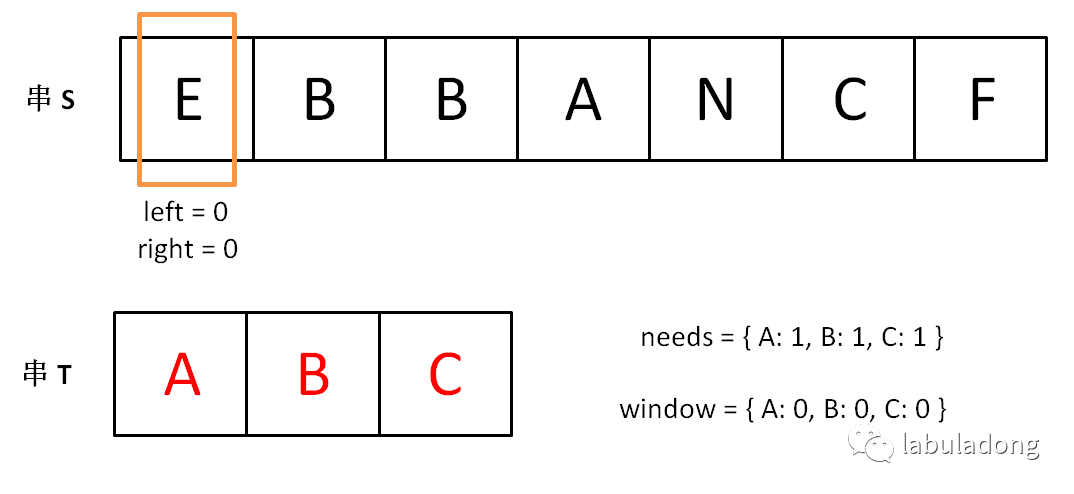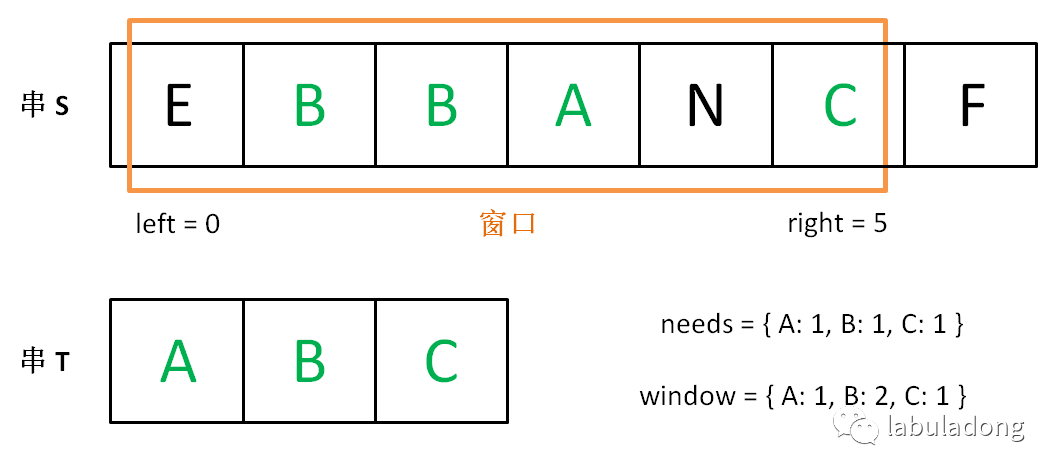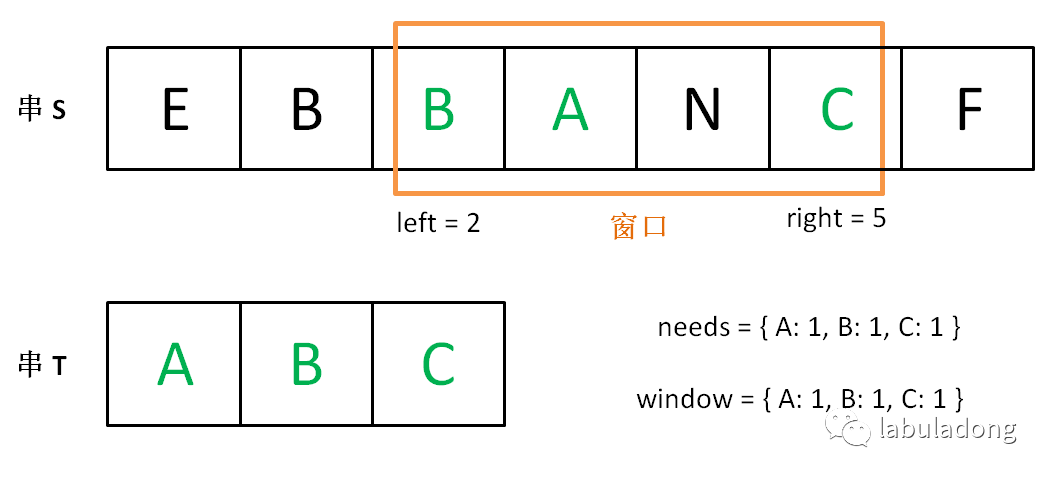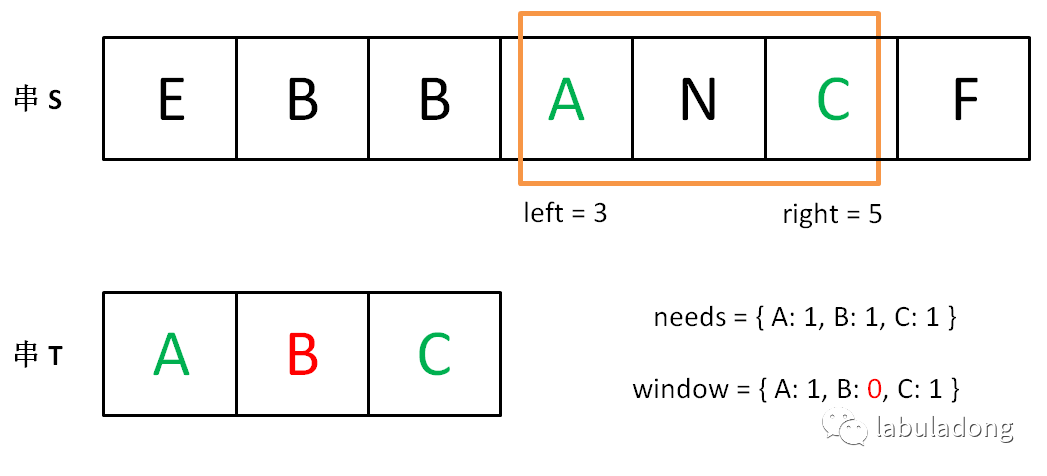# # 滑动窗口技巧

`unordered_map` 就是哈希表（字典），它的一个方法 count(key) 相当于 containsKey(key) 可以判断键 key 是否存在。

### # 一、最小覆盖子串``````for (int i = 0; i < s.size(); i++)
for (int j = i + 1; j < s.size(); j++)
if s[i:j] 包含 t 的所有字母:
更新答案
``````

1、我们在字符串 S 中使用双指针中的左右指针技巧，初始化 left = right = 0，把索引闭区间 [left, right] 称为一个「窗口」。

2、我们先不断地增加 right 指针扩大窗口 [left, right]，直到窗口中的字符串符合要求（包含了 T 中的所有字符）。

3、此时，我们停止增加 right，转而不断增加 left 指针缩小窗口 [left, right]，直到窗口中的字符串不再符合要求（不包含 T 中的所有字符了）。同时，每次增加 left，我们都要更新一轮结果。

4、重复第 2 和第 3 步，直到 right 到达字符串 S 的尽头。``````string s, t;
// 在 s 中寻找 t 的「最小覆盖子串」
int left = 0, right = 0;
string res = s;

while(right < s.size()) {
right++;
// 如果符合要求，移动 left 缩小窗口
while (window 符合要求) {
// 如果这个窗口的子串更短，则更新 res
res = minLen(res, window);
window.remove(s[left]);
left++;
}
}
return res;
``````

``````string s, t;
// 在 s 中寻找 t 的「最小覆盖子串」
int left = 0, right = 0;
string res = s;

// 相当于两个计数器
unordered_map<char, int> window;
unordered_map<char, int> needs;
for (char c : t) needs[c]++;

// 记录 window 中已经有多少字符符合要求了
int match = 0;

while (right < s.size()) {
char c1 = s[right];
if (needs.count(c1)) {
window[c1]++; // 加入 window
if (window[c1] == needs[c1])
// 字符 c1 的出现次数符合要求了
match++;
}
right++;

// window 中的字符串已符合 needs 的要求了
while (match == needs.size()) {
// 更新结果 res
res = minLen(res, window);
char c2 = s[left];
if (needs.count(c2)) {
window[c2]--; // 移出 window
if (window[c2] < needs[c2])
// 字符 c2 出现次数不再符合要求
match--;
}
left++;
}
}
return res;
``````

``````string minWindow(string s, string t) {
// 记录最短子串的开始位置和长度
int start = 0, minLen = INT_MAX;
int left = 0, right = 0;

unordered_map<char, int> window;
unordered_map<char, int> needs;
for (char c : t) needs[c]++;

int match = 0;

while (right < s.size()) {
char c1 = s[right];
if (needs.count(c1)) {
window[c1]++;
if (window[c1] == needs[c1])
match++;
}
right++;

while (match == needs.size()) {
if (right - left < minLen) {
// 更新最小子串的位置和长度
start = left;
minLen = right - left;
}
char c2 = s[left];
if (needs.count(c2)) {
window[c2]--;
if (window[c2] < needs[c2])
match--;
}
left++;
}
}
return minLen == INT_MAX ?
"" : s.substr(start, minLen);
}
``````

### # 二、找到字符串中所有字母异位词`How can this problem be marked as easy???`

``````vector<int> findAnagrams(string s, string t) {
// 用数组记录答案
vector<int> res;
int left = 0, right = 0;
unordered_map<char, int> needs;
unordered_map<char, int> window;
for (char c : t) needs[c]++;
int match = 0;

while (right < s.size()) {
char c1 = s[right];
if (needs.count(c1)) {
window[c1]++;
if (window[c1] == needs[c1])
match++;
}
right++;

while (match == needs.size()) {
// 如果 window 的大小合适
// 就把起始索引 left 加入结果
if (right - left == t.size()) {
res.push_back(left);
}
char c2 = s[left];
if (needs.count(c2)) {
window[c2]--;
if (window[c2] < needs[c2])
match--;
}
left++;
}
}
return res;
}
``````

### # 三、无重复字符的最长子串``````int lengthOfLongestSubstring(string s) {
int left = 0, right = 0;
unordered_map<char, int> window;
int res = 0; // 记录最长长度

while (right < s.size()) {
char c1 = s[right];
window[c1]++;
right++;
// 如果 window 中出现重复字符
// 开始移动 left 缩小窗口
while (window[c1] > 1) {
char c2 = s[left];
window[c2]--;
left++;
}
res = max(res, right - left);
}
return res;
}
``````

### # 最后总结

``````int left = 0, right = 0;

while (right < s.size()) {
right++;

while (valid) {
window.remove(s[left]);
left++;
}
}
``````